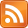SMS scnews item created by Daniel Daners at Fri 13 May 2016 2038
Type: Seminar
Distribution: World
Expiry: 16 May 2016
Calendar1: 16 May 2016 1400-1500
CalLoc1: AGR Carslaw 829
Auth: daners@d58-110-201-128.mas800.nsw.optusnet.com.au (ddan2237) in SMS-WASM

# Long time behavior of solutions of a reaction-diffusion equation on unbounded intervals with Robin boundary conditions

### Maolin Zhou

Maolin Zhou
University of New England, Armidale
16th May 2016 14:00-15:00, Carslaw Room 829 (AGR)

## Abstract

We study the long time behavior, as $t\to \infty$, of solutions of

$\begin{array}{llllllll}\hfill {u}_{t}& ={u}_{xx}+f\left(u\right),\phantom{\rule{2em}{0ex}}& \hfill & x>0,\phantom{\rule{1em}{0ex}}t>0,\phantom{\rule{2em}{0ex}}& \hfill & \phantom{\rule{2em}{0ex}}& \hfill & \phantom{\rule{2em}{0ex}}\\ \hfill u\left(0,t\right)& =b{u}_{x}\left(0,t\right),\phantom{\rule{2em}{0ex}}& \hfill & t>0,\phantom{\rule{2em}{0ex}}& \hfill & \phantom{\rule{2em}{0ex}}& \hfill & \phantom{\rule{2em}{0ex}}\\ \hfill u\left(x,0\right)& ={u}_{0}\left(x\right)\ge 0,\phantom{\rule{2em}{0ex}}& \hfill & x\ge 0,\phantom{\rule{2em}{0ex}}& \hfill & \phantom{\rule{2em}{0ex}}& \hfill & \phantom{\rule{2em}{0ex}}\end{array}$

where $b\ge 0$ and $f$ is an unbalanced bistable nonlinearity. By investigating families of initial data of the type ${\left\{\sigma \varphi \right\}}_{\sigma >0}$, where $\varphi$ belongs to an appropriate class of nonnegative compactly supported functions, we exhibit the sharp threshold between vanishing and spreading. More specifically, there exists some value ${\sigma }^{*}$ such that the solution converges uniformly to 0 for any $0<\sigma <{\sigma }^{*}$, and locally uniformly to a positive stationary state for any $\sigma >{\sigma }^{*}$. In the threshold case $\sigma ={\sigma }^{*}$, the profile of the solution approaches the symmetrically decreasing ground state with some shift, which may be either finite or infinite. In the latter case, the shift evolves as $Clnt$ where $C$ is a positive constant we compute explicitly, so that the solution is traveling with a pulse-like shape albeit with an asymptotically zero speed. Depending on $b$, but also in some cases on the choice of the initial datum, we prove that one or both of the situations may happen.

For Seminar announcements you can now subscribe to theSeminar RSS feed

Check also the PDE Seminar page. Enquiries to Daniel Hauer or Daniel Daners.

If you are registered you may mark the scnews item as read.
School members may try to .